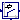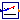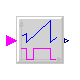## Buildings.Controls.Continuous

Package with models for discrete time controls

### Information

Extends from Modelica.Icons.VariantsPackage (Icon for package containing variants).

### Package Content

NameDescriptionLimPID P, PI, PD, and PID controller with limited output, anti-windup compensation and setpoint weightingPIDHysteresis PID controller with anti-windup, output limiter and output hysteresisPIDHysteresisTimer PID controller with anti-windup, hysteresis and timer to prevent short cyclingSignalRanker Ranks output signals such that y[i] >= y[i+1]NumberOfRequests Outputs the number of signals that are above/below a certain thresholdOffTimer Records the time since the input changed to falseExamples Collection of models that illustrate model use and test models

##Buildings.Controls.Continuous.LimPID

P, PI, PD, and PID controller with limited output, anti-windup compensation and setpoint weighting### Information

This model is identical to Modelica.Blocks.Continuous.LimPID except that it can be configured to have a reverse action.

If the parameter `reverseAction=false` (the default), then `u_m < u_s` increases the controller output, otherwise the controller output is decreased. Thus,

• for a heating coil with a two-way valve, set `reverseAction = false`,
• for a cooling coils with a two-way valve, set `reverseAction = true`.

Extends from Modelica.Blocks.Continuous.LimPID (P, PI, PD, and PID controller with limited output, anti-windup compensation and setpoint weighting).

### Parameters

TypeNameDefaultDescription
SimpleControllercontrollerTypeModelica.Blocks.Types.Simple...Type of controller
Realk1Gain of controller 
TimeTi Time constant of Integrator block [s]
TimeTd Time constant of Derivative block [s]
RealyMax1Upper limit of output
RealyMin0Lower limit of output
Realwp1Set-point weight for Proportional block (0..1)
Realwd0Set-point weight for Derivative block (0..1)
RealNi0.9Ni*Ti is time constant of anti-windup compensation
RealNd10The higher Nd, the more ideal the derivative block
BooleanreverseActionfalseSet to true for throttling the water flow rate through a cooling coil controller
Initialization
InitPIDinitTypeModelica.Blocks.Types.InitPI...Type of initialization (1: no init, 2: steady state, 3: initial state, 4: initial output)
BooleanlimitsAtInittrue= false, if limits are ignored during initializiation
Realxi_start0Initial or guess value value for integrator output (= integrator state)
Realxd_start0Initial or guess value for state of derivative block
Realy_start0Initial value of output

### Connectors

TypeNameDescription
input RealInputu_sConnector of setpoint input signal
input RealInputu_mConnector of measurement input signal
output RealOutputyConnector of actuator output signal

### Modelica definition

```block LimPID
"P, PI, PD, and PID controller with limited output, anti-windup compensation and setpoint weighting"
extends Modelica.Blocks.Continuous.LimPID(
yMin=0,
yMax=1);

parameter Boolean reverseAction = false
"Set to true for throttling the water flow rate through a cooling coil controller";
protected
parameter Real revAct = if reverseAction then -1 else 1;
end LimPID;
```

##Buildings.Controls.Continuous.PIDHysteresis

PID controller with anti-windup, output limiter and output hysteresis### Information

Block of a controller for set point tracking with a hysteresis element that switches the controller on and off.

If the controller is off, and the control error becomes larger than `eOn`, then the controller switches to on and remains on until the control error is smaller than `eOff`. When the controller is on, the set point tracking can be done using a P-, PI-, or PID-controller. In its off-mode, the control output is zero. Thus, the parameters `yMin` and `yMax` are used to constrain the output of the controller during its on mode only. This can be used, for example, to modulate a device between 0.3 and 1.0, and switch it to off when the control error is small enough.

Extends from Modelica.Blocks.Interfaces.SVcontrol (Single-Variable continuous controller).

### Parameters

TypeNameDefaultDescription
Hysteresis
RealeOn1if off and control error > eOn, switch to set point tracking
RealeOff-eOnif on and control error < eOff, set y=0
Booleanpre_y_startfalseValue of hysteresis output at initial time
Set point tracking
SimpleControllercontrollerTypeModelica.Blocks.Types.Simple...Type of controller
Realk1Gain of controller
TimeTi Time constant of Integrator block [s]
TimeTd Time constant of Derivative block [s]
RealyMax1Upper limit of output
RealyMin0Lower limit of output
Realwp1Set-point weight for Proportional block (0..1)
Realwd0Set-point weight for Derivative block (0..1)
RealNi0.9Ni*Ti is time constant of anti-windup compensation
RealNd10The higher Nd, the more ideal the derivative block
BooleanreverseActionfalseSet to true to enable reverse action (such as for a cooling coil controller)
Initialization
InitPIDinitTypeModelica.Blocks.Types.InitPI...Type of initialization (1: no init, 2: steady state, 3: initial state, 4: initial output)
BooleanlimitsAtInittrue= false, if limits are ignored during initializiation
Realxi_start0Initial or guess value value for integrator output (= integrator state)
Realxd_start0Initial or guess value for state of derivative block
Realy_start0Initial value of output

### Connectors

TypeNameDescription
input RealInputu_sConnector of setpoint input signal
input RealInputu_mConnector of measurement input signal
output RealOutputyConnector of actuator output signal

### Modelica definition

```model PIDHysteresis
"PID controller with anti-windup, output limiter and output hysteresis"
extends Modelica.Blocks.Interfaces.SVcontrol;

parameter Real eOn = 1
"if off and control error > eOn, switch to set point tracking";
parameter Real eOff = -eOn "if on and control error < eOff, set y=0";
parameter Boolean pre_y_start=false
"Value of hysteresis output at initial time";

parameter Modelica.Blocks.Types.SimpleController controllerType=Modelica.Blocks.Types.SimpleController.PID
"Type of controller";
parameter Real k=1 "Gain of controller";
parameter Modelica.SIunits.Time Ti "Time constant of Integrator block";
parameter Modelica.SIunits.Time Td "Time constant of Derivative block";
parameter Real yMax=1 "Upper limit of output";
parameter Real yMin=0 "Lower limit of output";
parameter Real wp=1 "Set-point weight for Proportional block (0..1)";
parameter Real wd=0 "Set-point weight for Derivative block (0..1)";
parameter Real Ni=0.9 "Ni*Ti is time constant of anti-windup compensation";
parameter Real Nd=10 "The higher Nd, the more ideal the derivative block";
parameter Boolean reverseAction = false
"Set to true to enable reverse action (such as for a cooling coil controller)";

parameter Modelica.Blocks.Types.InitPID initType=Modelica.Blocks.Types.InitPID.DoNotUse_InitialIntegratorState
"Type of initialization (1: no init, 2: steady state, 3: initial state, 4: initial output)";
parameter Boolean limitsAtInit=true
"= false, if limits are ignored during initializiation";
parameter Real xi_start=0
"Initial or guess value value for integrator output (= integrator state)";
parameter Real xd_start=0
"Initial or guess value for state of derivative block";
parameter Real y_start=0 "Initial value of output";

LimPID PID(
controllerType=controllerType,
k=k,
Ti=Ti,
yMax=yMax,
yMin=yMin,
wp=wp,
wd=wd,
Ni=Ni,
Nd=Nd,
initType=initType,
limitsAtInit=limitsAtInit,
xi_start=xi_start,
xd_start=xd_start,
y_start=y_start,
Td=Td,
reverseAction=reverseAction) "Controller for room temperature";
Modelica.Blocks.Logical.Hysteresis hys(
pre_y_start=pre_y_start,
uLow=eOff,
uHigh=eOn) "Hysteresis element to switch controller on and off";
Modelica.Blocks.Logical.Switch swi;
Modelica.Blocks.Sources.Constant zer(k=0) "Zero signal";
Modelica.Blocks.Math.Feedback feeBac;
Modelica.Blocks.Logical.Switch swi1;

equation
assert(eOff < eOn, "Wrong controller parameters. Require eOff < eOn.");
connect(zer.y, swi.u3);
connect(swi.y, y);
connect(u_m, PID.u_m);
connect(hys.y, swi.u2);
connect(PID.y, swi.u1);
connect(u_s, feeBac.u1);
connect(u_m, feeBac.u2);
connect(feeBac.y, hys.u);
connect(u_s, swi1.u1);
connect(hys.y, swi1.u2);
connect(u_m, swi1.u3);
connect(swi1.y, PID.u_s);
end PIDHysteresis;
```

##Buildings.Controls.Continuous.PIDHysteresisTimer

PID controller with anti-windup, hysteresis and timer to prevent short cycling### Information

Block of a controller for set point tracking with a hysteresis element that switches the controller on and off, and a timer that prevents the controller to short cycle.

The controller is similar to Buildings.Controls.Continuous.PIDHysteresis but in addition, it has a timer that prevents the controller from switching to on too fast. When the controller switches off, the timer starts and avoids the controller from switching on until `minOffTime` seconds elapsed.

Extends from Modelica.Blocks.Interfaces.SVcontrol (Single-Variable continuous controller).

### Parameters

TypeNameDefaultDescription
On/off controller
RealminOffTime600Minimum time that devices needs to be off before it can run again
RealeOn1if off and control error > eOn, switch to set point tracking
RealeOff-eOnif on and control error < eOff, set y=0
Booleanpre_y_startfalseValue of hysteresis output at initial time
Set point tracking
SimpleControllercontrollerTypeModelica.Blocks.Types.Simple...Type of controller
Realk1Gain of controller
TimeTi Time constant of Integrator block [s]
TimeTd Time constant of Derivative block [s]
RealyMax1Upper limit of modulating output
RealyMin0.3Lower limit of modulating output (before switch to 0)
Realwp1Set-point weight for Proportional block (0..1)
Realwd0Set-point weight for Derivative block (0..1)
RealNi0.9Ni*Ti is time constant of anti-windup compensation
RealNd10The higher Nd, the more ideal the derivative block
BooleanreverseActionfalseSet to true to enable reverse action (such as for a cooling coil controller)
Initialization
InitPIDinitTypeModelica.Blocks.Types.InitPI...Type of initialization (1: no init, 2: steady state, 3: initial state, 4: initial output)
BooleanlimitsAtInittrue= false, if limits are ignored during initializiation
Realxi_start0Initial or guess value value for integrator output (= integrator state)
Realxd_start0Initial or guess value for state of derivative block
Realy_start0Initial value of output

### Connectors

TypeNameDescription
input RealInputu_sConnector of setpoint input signal
input RealInputu_mConnector of measurement input signal
output RealOutputyConnector of actuator output signal
output RealOutputtOnTime since boiler switched on
output RealOutputtOffTime since boiler switched off
output BooleanOutputonOutputs true if boiler is on

### Modelica definition

```model PIDHysteresisTimer
"PID controller with anti-windup, hysteresis and timer to prevent short cycling"
extends Modelica.Blocks.Interfaces.SVcontrol;

parameter Real minOffTime=600
"Minimum time that devices needs to be off before it can run again";
parameter Real eOn = 1
"if off and control error > eOn, switch to set point tracking";
parameter Real eOff = -eOn "if on and control error < eOff, set y=0";
parameter Boolean pre_y_start=false
"Value of hysteresis output at initial time";

parameter Modelica.Blocks.Types.SimpleController controllerType=Modelica.Blocks.Types.SimpleController.PID
"Type of controller";
parameter Real k=1 "Gain of controller";
parameter Modelica.SIunits.Time Ti "Time constant of Integrator block";
parameter Modelica.SIunits.Time Td "Time constant of Derivative block";
parameter Real yMax=1 "Upper limit of modulating output";
parameter Real yMin=0.3
"Lower limit of modulating output (before switch to 0)";
parameter Real wp=1 "Set-point weight for Proportional block (0..1)";
parameter Real wd=0 "Set-point weight for Derivative block (0..1)";
parameter Real Ni=0.9 "Ni*Ti is time constant of anti-windup compensation";
parameter Real Nd=10 "The higher Nd, the more ideal the derivative block";
parameter Boolean reverseAction = false
"Set to true to enable reverse action (such as for a cooling coil controller)";

parameter Modelica.Blocks.Types.InitPID initType=Modelica.Blocks.Types.InitPID.DoNotUse_InitialIntegratorState
"Type of initialization (1: no init, 2: steady state, 3: initial state, 4: initial output)";
parameter Boolean limitsAtInit=true
"= false, if limits are ignored during initializiation";
parameter Real xi_start=0
"Initial or guess value value for integrator output (= integrator state)";
parameter Real xd_start=0
"Initial or guess value for state of derivative block";
parameter Real y_start=0 "Initial value of output";

Modelica.Blocks.Interfaces.RealOutput tOn "Time since boiler switched on";
Modelica.Blocks.Interfaces.RealOutput tOff "Time since boiler switched off";

LimPID con(
controllerType=controllerType,
k=k,
Ti=Ti,
Td=Td,
wp=wp,
wd=wd,
Ni=Ni,
Nd=Nd,
initType=initType,
limitsAtInit=limitsAtInit,
xi_start=xi_start,
xd_start=xd_start,
y_start=y_start,
final yMin=yMin,
final yMax=yMax,
reverseAction=reverseAction) "Controller to track setpoint";
protected
Modelica.Blocks.Sources.Constant zer(k=0) "Zero signal";
Modelica.Blocks.Logical.Switch switch2;
Modelica.Blocks.Logical.GreaterEqualThreshold greaterEqualThreshold(threshold=
minOffTime);
Modelica.Blocks.Logical.And and3;
public
OffTimer offHys;
Modelica.Blocks.Logical.Timer onTimer;
protected
Modelica.Blocks.Logical.Not not1;
public
Modelica.Blocks.Logical.Timer offTimer;
Modelica.Blocks.Interfaces.BooleanOutput on
"Outputs true if boiler is on";
protected
Modelica.Blocks.Logical.Switch switch1;
public
Modelica.Blocks.Math.Feedback feeBac;
Modelica.Blocks.Logical.Hysteresis hys(
pre_y_start=pre_y_start,
uLow=eOff,
uHigh=eOn) "Hysteresis element to switch controller on and off";
equation
connect(u_m, con.u_m);
connect(zer.y,switch2. u3);
connect(switch2.y, y);
connect(and3.y,switch2. u2);
connect(greaterEqualThreshold.y, and3.u1);
connect(offHys.y, greaterEqualThreshold.u);
connect(con.y,switch2. u1);
connect(onTimer.y, tOn);
connect(offTimer.y, tOff);
connect(and3.y, on);
connect(and3.y, onTimer.u);
connect(and3.y, not1.u);
connect(not1.y, offTimer.u);
connect(and3.y, switch1.u2);
connect(u_s, switch1.u1);
connect(u_m, switch1.u3);
connect(switch1.y, con.u_s);
connect(u_s, feeBac.u1);
connect(u_m, feeBac.u2);
connect(feeBac.y, hys.u);
connect(hys.y, offHys.u);
connect(hys.y, and3.u2);
end PIDHysteresisTimer;
```

##Buildings.Controls.Continuous.SignalRanker

Ranks output signals such that y[i] >= y[i+1]### Information

Block that sorts the input signal `u[:]` such that the output signal satisfies `y[i] >= y[i+1]` for all `i=1, ..., nin-1`.

This block may for example be used in a variable air volume flow controller to access the position of the dampers that are most open.

Extends from Modelica.Blocks.Interfaces.MIMO (Multiple Input Multiple Output continuous control block).

### Parameters

TypeNameDefaultDescription
Integernin1Number of inputs
IntegernoutninNumber of outputs

### Connectors

TypeNameDescription
input RealInputu[nin]Connector of Real input signals
output RealOutputy[nout]Connector of Real output signals

### Modelica definition

```block SignalRanker "Ranks output signals such that y[i] >= y[i+1]"
extends Modelica.Blocks.Interfaces.MIMO(final nout=nin);
protected
Real t "Temporary variable";
algorithm
y[:] := u[:];
for i in 1:nin loop
for j in 1:nin-1 loop
if y[j] < y[j+1] then
t      := y[j+1];
y[j+1] := y[j];
y[j]   := t;
end if;
end for;
end for;
end SignalRanker;
```

##Buildings.Controls.Continuous.NumberOfRequests

Outputs the number of signals that are above/below a certain threshold### Information

Block that outputs the number of inputs that exceed a threshold. The parameter `kind` is used to determine the kind of the inequality. The table below shows the allowed settings.

Value of parameter `kind` Output signal incremented by 1 for each i ∈ {1, ..., nin} if
0 `u[i] > threShold`
1 `u[i] ≥ threShold`
2 `u[i] ≤ threShold`
3 `u[i] < threShold`

This model may be used to check how many rooms exceed a temperature threshold.

Extends from Modelica.Blocks.Interfaces.BlockIcon (Basic graphical layout of input/output block).

### Parameters

TypeNameDefaultDescription
Integernin Number of inputs
RealthreShold0Threshold
Integerkind Set to 0 for u>threShold, to 1 for >=, to 2 for <= or to 3 for <

### Connectors

TypeNameDescription
output IntegerOutputyNumber of input signals that violate the threshold
input RealInputu[nin]Input signals

### Modelica definition

```block NumberOfRequests
"Outputs the number of signals that are above/below a certain threshold"
extends Modelica.Blocks.Interfaces.BlockIcon;

parameter Integer nin "Number of inputs";
parameter Real threShold = 0 "Threshold";
parameter Integer kind
"Set to 0 for u>threShold, to 1 for >=, to 2 for <= or to 3 for <";
Modelica.Blocks.Interfaces.IntegerOutput y
"Number of input signals that violate the threshold";
Modelica.Blocks.Interfaces.RealInput u[nin] "Input signals";
algorithm
y := 0;
for i in 1:nin loop
if kind == 0 then
if u[i] > threShold then
y := y+1;
end if;
end if;
if kind == 1 then
if u[i] >= threShold then
y := y+1;
end if;
end if;
if kind == 2 then
if u[i] <= threShold then
y := y+1;
end if;
end if;
if kind == 3 then
if u[i] < threShold then
y := y+1;
end if;
end if;
end for;
end NumberOfRequests;
```

##Buildings.Controls.Continuous.OffTimer

Records the time since the input changed to false### Information

Block that records the time that has elapsed since its input signal switched to false.

At the beginning of the simulation, this block outputs the time that has elapsed since the start of the simulation. Afterwards, whenever its input switches to false, the timer is reset.

Extends from Modelica.Blocks.Interfaces.partialBooleanBlockIcon (Basic graphical layout of logical block).

### Connectors

TypeNameDescription
input BooleanInputuConnector of Boolean input signal
output RealOutputyConnector of Real output signal

### Modelica definition

```model OffTimer "Records the time since the input changed to false"
extends Modelica.Blocks.Interfaces.partialBooleanBlockIcon;

Modelica.Blocks.Interfaces.BooleanInput u "Connector of Boolean input signal";
Modelica.Blocks.Interfaces.RealOutput y "Connector of Real output signal";

protected
discrete Modelica.SIunits.Time entryTime "Time instant when u became true";
initial equation
pre(entryTime) = 0;
equation
when (not u) then
entryTime = time;
end when;
y = time - entryTime;
end OffTimer;
```

Automatically generated Thu Oct 24 15:08:04 2013.